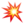/javascript

# Top 10 JavaScript Array tricks

These are some of the very basic Javascript methods that will help you get better in Javascript. Let’s straightly jump into coding..## Fill array with data

``````var myArray = new Array(10).fill('A');
console.log(myArray);

//Output
['A', 'A', 'A', 'A', 'A', 'A', 'A', 'A', 'A', 'A'];``````

Every now and then we need to fill some array with any data. Here we are making use of the `fill` method from the Array API. Notice the count `10`, it denotes the number of times to fill the content specified inside the `fill()` function.

## Merge Arrays

``````var bikes = ['TVS', 'BMW', 'Ducati'];
var cars = ['Mercedes', 'Ford', 'Porsche'];
var autoMobiles = [...bikes, ...cars];
console.log(autoMobiles);

//Output
['TVS', 'BMW', 'Ducati', 'Mercedes', 'Ford', 'Porsche'];``````

Merging arrays are a major use case in any application that we develop. We might have user array and a permission array denoting permissions for the user. We might need to combile it as a array in order to perform something on the API. Here we are making use of the `spread` operator `...` to combine or merge arrays.

## Intersection of arrays

``````var setA = [5, 10, 4, 7, 1, 3];
var setB = [3, 11, 1, 10, 2, 6];
var duplicatedValues = [...new Set(setA)].filter((x) => setB.includes(x));
console.log(duplicatedValues);

//Output
[10, 1, 3];``````

This is a crucial use case. Imagine a use case where where you need to find out the duplicated values from two arrays, or also called as intersection. We are first creating a `unique` array by combining the `...` spread operator and `Set` function and then filter if the other array has those values.

## Remove duplicates

``````var mixedArray = [12, 'web development', '', NaN, undefined, 0, true, false];
var whatIsTrue = mixedArray.filter(Boolean);
console.log(whatIsTrue);

//Output
[12, 'web development', true];``````

We might need to filter falsy values like `[0, undefined, null, false]`. This is a simple trick to do so.

## Get random value

``````var numbers = [];
for (let i = 0; i < 10; i++) {
numbers.push(i);
}

var random = numbers[Math.floor(Math.random() * numbers.length)];
console.log(random);

//Output
4;``````

Need a random integer value ? No problem. In one line of code you can do so.

## Reverse an Array

``````var reversedArray = numbers.reverse();
console.log(reversedArray);

//Output
[9, 8, 7, 6, 5, 4, 3, 2, 1, 0];``````

These are just very basic built in functions to reverse the given array.

## Sum of all values in array

``````var sumOfAllNumbers = numbers.reduce((x, y) => x + y);
console.log(sumOfAllNumbers);

//Output
45;``````

## Remove duplicates from array

``````var duplicatedArray = ['hello', 'hello', 'web developers'];
var nonDuplicatedArray = [...new Set(duplicatedArray)];
console.log(nonDuplicatedArray);

//Output
['hello', 'web developers'];``````

This is a super efficient way to remove duplicates from array. We combine `...` spread operator and the `Set()` API, to achieve it.

Thanks for reading. I hope this gave you an some insights about the Javascript array methods. Keep following and Stay tuned for more amazing blogs.#### HariHaran

Full Stack Developer